Learning Library

Color by Number Subtraction

Help make two-digit subtraction a little more colorful for your second grader with these color by number worksheets.

Click on a worksheet in the set below to see more info or download the PDF.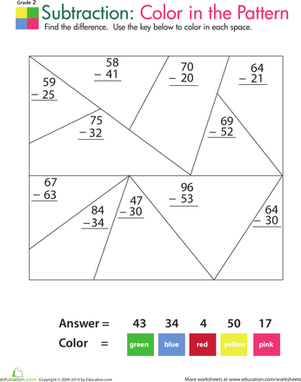Color by Number: Practice Two-Digit Subtraction 1

Is your child tired of the usual math worksheets? Modeled after classic color by number pages, this subtraction worksheet makes finding the difference fun!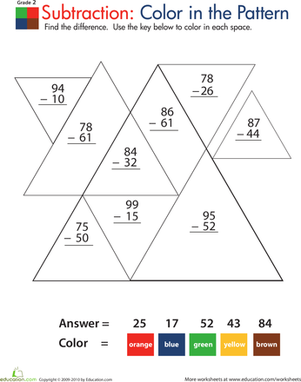Color by Number: Practice Two-Digit Subtraction 2

Modeled after color by number coloring pages, this math worksheet makes subtraction fun! Your child will enjoy coloring to reveal a pretty geometric pattern.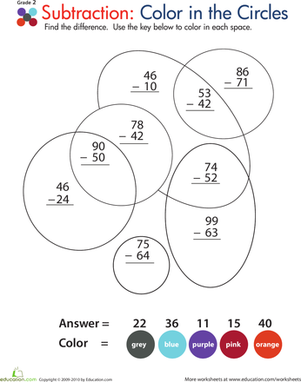Color by Number: Practice Two-Digit Subtraction 3

Part color by number coloring page, part math worksheet, this second grade printable have your child begging to practice subtraction!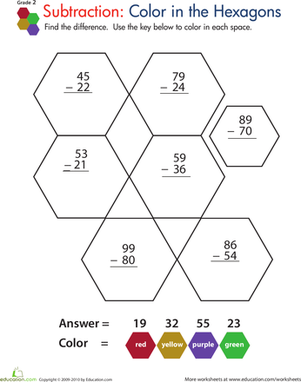Color by Number: Practice Two-Digit Subtraction 4

Subtraction is fun in this second grade math worksheet modeled after classic color by number pages.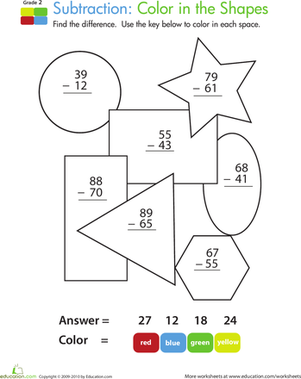Color by Number: Practice Two-Digit Subtraction 5

Doubling as a color by number coloring page, this second grade math worksheet makes practicing two-digit subtraction a blast!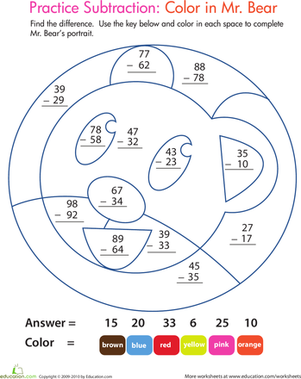Color by Number: Practice Two-Digit Subtraction 6

This math worksheet is just the thing to entice your child to practice subtraction. Once he's solved the two-digit subtraction problems, he'll color Mr. Bear.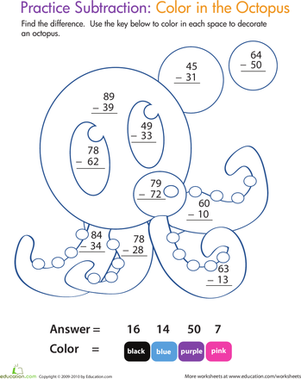Color by Number: Practice Two-Digit Subtraction 7

Doubling as a color by number coloring page, this second grade math worksheet makes practicing two-digit subtraction fun and enriching at the same time!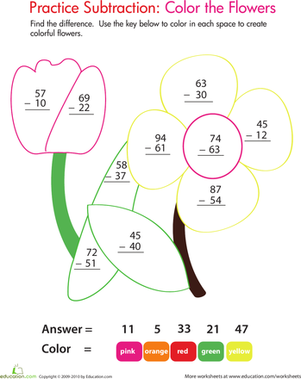Color by Number: Practice Two-Digit Subtraction 8

This subtraction color by number worksheet offers both math enrichment and a coloring activity. Second graders solve two-digit problems, then color!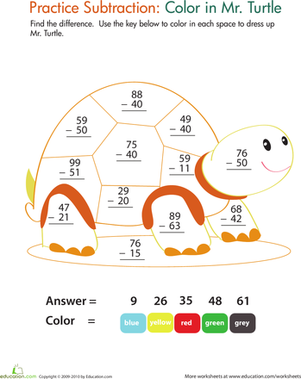Color by Number: Practice Two-Digit Subtraction 9

Doubling as a color by number coloring page, this 2nd grade worksheet makes practicing two-digit subtraction fun without skimping on the math help.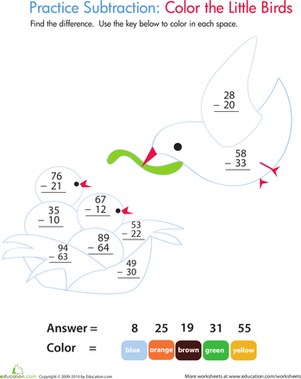Color by Number: Practice Two-Digit Subtraction 10

Doubling as a color by number coloring page, this 2nd grade math worksheet makes practicing two-digit subtraction fun.

Want to download this whole set as a single PDF?

Create new collection

0

New Collection>

0 items

What could we do to improve Education.com?

Please note: Use the Contact Us link at the bottom of our website for account-specific questions or issues.

What would make you love Education.com?